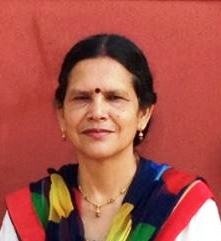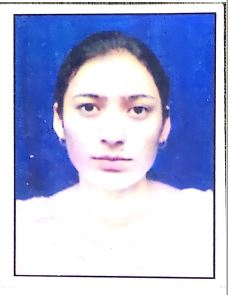2(f),12(B) Status

Estd.- 1976

# Lalit Sen Institute Of Business Education & Technology

A Post-Graduate Institute Affiliated to HPU, Shimla/SPU, Mandi

# Mathematics Department

Scope of Mathematics
Mathematics is perhaps the biggest discovery of all times. Maths has immense scope for a variety of fields of study and professions.

A strong background in Maths is required for areas as diverse as Engineering, Information Technology, Physical Sciences, Statistics, Astronomy, Economics, Banking and Accounting, Insurance, MBA, MCA among others.

Job Opportunities for Maths Enthusiasts
Academia (teaching and research), Chartered Accountant, Banking, Computer System Analyst, Software Engineering, Actuary, Investment Analyst, Data Analyst, Systems Analyst, Financial Manager, Meteorologist.

A strong grounding in numbers opens the door to a wealth of opportunities!

### PROGRAMME SPECIFIC OUTCOMES OF B.Sc. AND B.A. MATH

PSO-1 On the successful completion of the course, the graduates understand the concepts of calculus (Differential and integral, differential equations (ordinary and partial, algebra (abstract, linear) and analysis (real, complex and numerical) and mechanics.
PSO-2 Read, understand and construct correct mathematical proofs
PSO-3 Develop power of reasoning, critical thinking, problem solving ability, developing new ideas, drawing logical conclusions and high level of numeracy.
PSO-4 Explains the importance of Maths and its techniques to solve real life problems.
PSO-5 Able to communicate effectively both orally and in writing.
PSO-6 Develop skills in analyzing and interpreting data.
PSO-7 Graduates of maths programme will be able to apply their knowledge in modern industry, teaching and other fields such as MBA, MCA, Mathematical Computing and research.
PSO-8 Studying maths simply opens the doors to a wealth of opportunities.

### MATHEMATICS (B.Sc. PHYSICAL SCIENCE) STRUCTURE

Paper Code Paper Title Credit ESE CCA Max.
Mark
Exam.
Duration
Hours

First Semester

Core course-1

MATH101TH

MATH101IA

Differential Calculus

6

70

30

100

3

Ability Enhancement Course – I (Compulsory)

English/Hindi(Communication) Environmental Science

4

80

20

100

3

Total

10

200

Second semester

Core course-2

MATH201TH

MATH201IA

Differential Equations

6

70

30

100

3

Ability

Enhancement

Course-2

(Compulsory)

English/Hindi(Communication) Environmental Science

4

80

20

100

3

Total

10

200

Third semester

Core course-3

MATH101TH

MATH101IA

Real Analysis

6

70

30

100

3

SEC-I

MATH302TH

OR

MATH303TH

OR

MATH304TH

Logic And Sets

OR

Analytical Geometry

OR

Integral Calculus

4

70

30

100

3

Total

10

200

Fourth Semester

Core course-4

MATH401TH

MATH401IA

Algebra

6

70

30

100

3

Skill Enhancement Course -2

MATH402TH

OR

MATH403TH

OR

MATH404TH

Vector Calculus

Or

Theory of Equations

Or

Number Theory

4

70

30

100

3

Total

10

200

Fifth semester

Discipline Specific elective Course-1

MATH501TH

OR

MATH502TH

OR

MATH503TH

Matrices

OR

Mechanics

OR

Linear  Algebra

6

70

30

100

3

Skill Enhancement Course -3

MATH504TH

OR

MATH505TH

OR

MATH506TH

Mathematical Finance

OR

Mathematical

Modeling

4

70

30

100

3

Total

10

200

Sixth Semester

Discipline Specific elective Course-2

MATH601TH

Or

MATH602TH

Or

MATH603TH

Numerical Methods

OR

Complex Analysis

OR

Linear programming

6

70

30

100

3

Skill Enhancement Course -4

MATH604TH

MATH605TH

OR

MATH606TH

Boolean Algebra

OR

Transportation And

Game Theory

OR

Graph  Theory

4

70

30

100

3

Total

10

200

### B.Sc. COURSE WISE OUTCOMES

Paper Code Paper Title Course outcome

MATH101TH

CORE COURSE-1

Differential calculus

·      Use of e - δ definition to find the limits and continuity of functions.

·      Study relationship between continuation and differentiation.

·      Able to understand the  idea of limits of functions by  L- hospitals rule

·      Learn the proofs of general theorem and their geometrical consequences.

·      Learns the notation of concavity convexity asymptotes.

·      Are able to trace the curves in polar and rectangular coordinates.

·      Understands the concepts of limit and continuity of functions of several variables.

·      Are able to convert Cartesian to polar coordinates and vice versa.

·      Learn the proof and applications of Euler’s theorem on homogeneous functions

·      Are able to calculate Jacobeans’.

Ability Enhancement

Compulsory Course -I

English/Hindi(Communication)/Environmental Science

These courses are the courses based upon the content that leads to Knowledge enhancement. And are value-based and/or skill-based and are aimed at providing hands-on-training, competencies, skills etc. in English and/Hindi and Environment science.

MATH201TH

CORE COURSE -2

Differential equations

·      Understands basic definitions and terminology associated with ordinary differential equations.

·      Learn rules to find integrating factors.

·      Distinguish between linear and non linear, ordinary and partial differential equations.

·      Recognize and solve homogeneous and non homogeneous equations by different methods

·      Students are able to apply the methods of variation of parameters and reduction of order.

·      Use working to determine linear dependence and linear independent of functions.

·      Learns to solve Cauchy Euler’s equations.

·      Learn the concepts of total differential equations.

·      Can form partial differential equations of first order and use Langrange’s methods to solve partial differential equations.

·      Are able to find solutions of partial differential equations by Charpit’s method.

·      Learn to classify second order partial differential equation through lustration only.

Ability Enhancement

Compulsory Course -II

English/Hindi(Communication)/Environmental Science

These courses are the courses based upon the content that leads to Knowledge enhancement. And are value-based and/or skill-based and are aimed at providing hands-on-training, competencies, skills etc. in English and/Hindi and Environment science.

MATH301TH  CORE COURSE -3

REAL ANALYSIS

·      Define and recognize sequence and series of real numbers and their conversions and uniform conversions

·      Recognize the difference between point wise conversions and uniform conversions of sequence of function.

·      Use comparison, condensation, ratio, root condensation and Leibnitz’s test for conversion of series.

·      Construct mathematical proofs of basic results in real analysis

·      are able to comprehend bounded sets ,Archimedean properties and Bolzano-Weierstrass theorem

·      Students can produce proofs of results of real analysis.

Skill Enhancement Course-1

MATH302TH

OR

MATH303T

OR

MATH304TH

Analytical Geometry

OR

Integral Calculus

Integral Calculus, (MATH304TH)

·         Learns integration by partial functions and properties of the definite integrals

·         Establish various reduction formulae

·         Are able to connect to integrals (smaller index+1)

·         Learn to find area and length of curves in planes.

·      Evaluate double and triple integrals.;

MATH401TH  CORE COURSE-4

Algebra

·      Study the definition of groups rings and fields.

·      Are able to understand concept if subgroups, normal subgroups and quotient groups.

·      Use concepts of homomorphism isomorphism and endomorphism for group sand rings.

·      Use canonical types of groups like cyclic groups ,permutation groups and rings such as polynomial rings and quotient  rings

·      Are able to find cossets and related theorem.

·      Produce groups of theorem on algebra.

Skill Enhancement Course-2

MATH402TH

OR

MATH403TH

Vector Calculus

OR

Theory of Equations

OR

Number Theory

VECTOR CALCULUS(MATH402TH

·       Study differentiation & partial differentiation of a vector sum.

·       Learn the concept of the dot product.

·       To study to find gradient, divergence and curl of vectors.

(Theory of Equations MATH403TH)

·      Students will be familiar with general properties of polynomials and their graphical representation.

·      Will be able to compute maximum and minimum values of polynomials.

·      Will gain the ability to use Descartes role of science for positive and negative roots.

·      Will come to know about the concept of symmetric functions.

·      Will use transformation of equations and will be able to find solutions of binomial and reciprocal equations.

·      Students will gain the ability to find algebraic solutions of cubic and biquadrate’s.

DSE-Course-1

MATH501TH

OR

MATH502TH

OR

MATH503TH

Matrices

OR

Mechanics

OR

Linear  Algebra

Linear  Algebra(MATH503TH)

·         Analysis vectors in Rn algebraically

·         Learn the concepts of linear independence and dependence, linear span basis and dimensions.

·         Study vector spaces and subspaces.

·         Use matrix algebra and relate matrices to linear transformations.

·         Study inner product spaces and Cauchy Schwarz’s in equality.

Skill Enhancement Course -3

MATH504TH

OR

MATH505TH

OR

MATH506TH

Probability and Statistics

OR

Mathematical Finance

OR

Mathematical Modeling

DSE Course- 2

MATH601TH

OR

MATH602TH

OR

MATH603TH

Numerical analysis

OR

Complex methods

OR

Analysis

Linear programming

Numerical Analysis,(MATH601th)

·         Study to find the approximate rules of non linear equation s by using different methods such as bisection, secant and Newton Repson method.

·         How to find missing numbers from the available data and the estimate value of known quantity between the two known quantities.

·          To find the value of definite integral from set of tabulated values of the integrand by using trapezoidal and Simpsons rule.

Complex Methods (MATH602TH)

·         Define functions in their complex domains

·         Study relation between points in Cartesian plane and complex planes

·         Study the complex integration of functions, limit and continuity of complex functions, Cauchy Riemann equation harmonic functions.

Skill Enhancement Course--IV

MATH604TH

Or

MATH605TH

Or

MATH606TH

Boolean Algebra

Or

Transportation And

Game Theory

Or

Graph  Theory

Boolean algebra (MATH604TH)

·           Study the definition, examples of ordered sets, maps between the ordered sets, maximal and minimal elements,l attices, sublattices and homomorphisms.

·           Definition and properties of modular and distributive lattices, boolian polynomials , minimal form of boolian polynomials.

### Mechanism of communication

• Lectures are delivered prmerly through chalk and talk.
• Tutorial suplliment the lecture mby providing exercises and example problems to enhance the understanding.
• Written assignments , two class tests ,asking questions in between the lectures ,participation in classroom,discussions and minor tests (onje) conducted by nthe college gives assessment of thr students understanding of the course.Name : Ms. Renu Kalia (H.O.D.)

Email : renukalia65@gmail.com

Mobile No. : 9418666966

Qualification : M.A., M.Phil.Name : Ms. Manjula Thakur

Email : manjulathakur_2007@hotmail.com

Mobile No. : 9418747084

Qualification : M.Sc., M .Phil.Name : Dr. Shaloo Devi

Email : shaloosharma2673@gmail.com

Mobile No. : 9996294503

Qualification : M.Sc., M .Phil., Ph.D.

#### Pi Day Celebration 2020

Subject Syllabus
Mathematics Click for Syllabus

### Our AchievementsPioneer institution to start courses under Self financing scheme in the state of Himachal Pradesh.First college in the state to introduce the concept of B. Ed. under self financing scheme.First college in the state to introduce M. Sc’s in Physics and Chemistry.Has consistently maintained a tradition of excellence in sports and culture for the last 40 years.Designated as Centre of Excellence for Boxing by the District Sports Council, Mandi.Presently has 17 departments with a student strength of more than 3000.